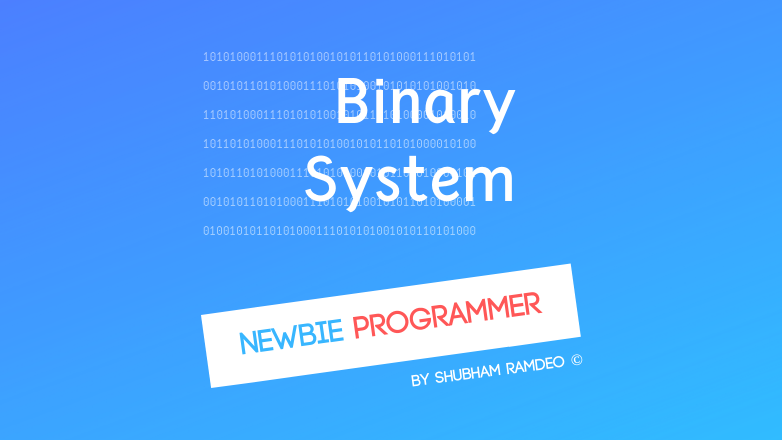# Binary Number System

PUBLISHED Sep 3rd, 2015

That’s how the computer talks. Can you understand Binary Number System ? zeros and ones !This is again a miscellaneous post, fun to write about but it’s very useful for any computer Geek. We have discussed so many times that Computer cannot understand stuffs like 1,2,3,… or A,B,C… etc, All it can understand is ones and zeros. That means that whatever we have a meaning for is translated into a series of zeros and ones. Called as Binary Numbers. Actually I am about to write about Bitwise Operators at my Newbie Programmer Series but before jumping we need to learn some basics about the Binary Number

The number system We Use is called as Decimal System. We sometimes also use Roman System. Computer has its own language which consists of zeros and ones which is called as Binary System. There are many other too, Hexadecimal, Octal etc But our focus is about Binary only.

Binary means two. As we have only two characters, zero and one to write.

There is one more meaning to binary system and that is that we use the Powers of ‘2’ to describe all the numbers.

The part a geek is concentrated about is not the history of this system but how to play around with it and so I am. Let us learn how to convert a number into binary or vice versa.

### How to Convert a number ( decimal ) into Binary System ?

It’s very simple. Start dividing the number by 2. If you got an even reminder, write a 0, if you got an odd reminder write 1 and keep dividing by 2 until the division ends. Then reverse the sequence of reminders. So for example, lets we want to convert 144 into binary form.

So we divide it by 2:

``````2|144
2|72    got reminder 0
2|36    got reminder 0
2|18    got reminder 0
2|9     got reminder 0
2|4     got reminder 1
2|2     got reminder 0
2|1     got reminder 0
2|0     got reminder 1
``````

Now on reversing the reminder sequence, we get:

144 = 10010000

Lets do one more. Lets convert 41 into binary. So on repeated dividing by 2:

``````2|41
2|20    got reminder 1
2|10    got reminder 0
2|5     got reminder 0
2|2     got reminder 1
2|1     got reminder 0
2|0     got reminder 1
``````

So we get:

41 = 101001

Isn’t that cool ?!?!?!?!

Lets do the reverse.

### How to Convert a Binary Number into Decimal ?

As I have written above that binary system involves powers of ‘2’. So all you need is that you just remember this simple sequence BOX of Powers of ‘2’ :

27   26   25   24   23   22   21   20

=

ie.   128    64   32   16    8     4     2     1

Now arrange the given binary number. The 1s would mean to turn those number on and 0s means to turn them off.

So as we did above, to convert  1001000 back to decimal, Just arrange them in that sequence. The number where there is 1 should be added and those with 0s should be skipped. As the given binary number is 7 digit long, so sequence up to 27  i.e. 128 is enough.

``````128   64    32    16    8    4    2    1
1     0     0     1    0    0    0    0
``````

128 + 16

= 144

So I simply added the numbers which were turned ‘on’ by the binary number.

Lets do another one. 101001. Its 6 digit long so sequence up to 26 i.e. 32 is enough so :

``````  32   16    8    4    2    1
1     0     1    0    0    1
``````

32 + 8 + 1 = 41

Isn’t that cool to ?!?!?!?!

Anyways that’s all we need to learn about basic Binary System. In the next miscellaneous post, we will do Mathematical Reasoning, One of my favorite topics in maths that will show you how logic is possible with maths ! So stay connected.

#### You might also like:

(prev)
(next)

(rand) Structure some Examples
(rand) Mathematical Reasoning
(rand) Introduction to Computer